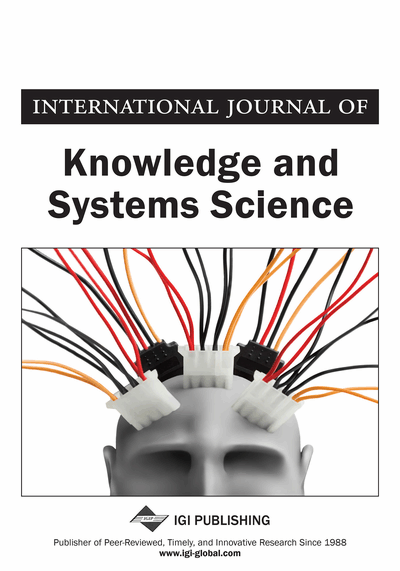# Particle Swarm Optimization For Hidden Markov Model

Nabil M. Hewahi (Department of Computer Science, University of Bahrain, Zallaq, Bahrain)
DOI: 10.4018/IJKSS.2015040101
Available
\$37.50
No Current Special Offers

## Abstract

Hidden Markov Model (HMM) is a very well known method as a statistical model used for intelligent systems applications. Due to its involvement in various applications, it would be very important to have a good representation of HMM for the given problem to achieve good results. In this paper, we propose a theoretical approach that can be followed to obtain the best structure of HMM based on Particle Swarm Optimization (PSO) concepts. Given a set of comprehensive visible and invisible states, we propose a method based on PSO concepts to evolve an optimum HMM structure design. The proposed approach deals with two factors related to HMM, generating new states and updating probability values. The main steps followed in the proposed approach involve three main phases, the first phase is generating randomly a population of HMMs, the second phase is converting the generated HMM to PSO required format and the third phase is the application of PSO to find out the optimum HMM . The importance of the proposed approach over other previous approaches is that other approaches deal only with probability updating.
Article Preview
Top

## 1. Introduction

Hidden Markov Model (HMM) is a statistical model based on probabilities used in various applications such as cryptanalysis, machine translation, speech and hand recognition, natural language processing, gene prediction and bioinformatics (Allison, L. Stern, L., Edgoose, T., and Dix, T., 2000; Furui, S., and Itoh, D., 2001; Gunter, S., and Bunke, H., 2002; Hassan, M., Nath, B. and Kirley, M., 2007; Hewahi, N., 2010; Li, J., Najmi, A., and Gray, R., 2000; Rabiner, L., 1989; Seymore, K., McCallum, A., and Rosenfeld, R., 1999; Soruri, M., Hamid, S., and Sadr, J., 2013; Stern, L., Allison, L., Coppel, R., and Dix, T., 2001). A Markov model is a probabilistic process over a finite set, {S1, ..., Sk}, usually called its states. Each state-transition generates a character from the alphabet of the process. In a regular Markov model, the state is directly visible to the observer, and therefore the state transition probabilities are the only parameters. In a hidden Markov model, the state is not directly visible, but variables influenced by the state are visible. Each state has a probability distribution over the possible output tokens. Therefore, the sequence of tokens generated by a HMM gives some information about the sequence of states. There are three problems associated with HMM, evaluation, decoding, and learning problems. The evaluation problem, given the parameters of the model, compute the probability of a particular output sequence, and the probabilities of the hidden state values given that output sequence. The evaluation problem can be solved by forward-backward algorithm. The decoding problem, given the parameters of the model, find the most likely sequence of hidden states that could have generated a given output sequence. This is solved by Viterbi algorithm. The learning problem, given an output sequence or a set of such sequences, find the most likely set of state transition and output probabilities. This problem is solved by the Baum-Welch algorithm ( Abraham, A., Guo, H., and Liu, H., 2006; Rabiner, L., 1989).

Gunter and Bunke (Gunter, S., and Bunke, H., 2002) combined various HMMs at a more elementary level and shown that this sort of combination improves the system's performance. Another application is using HMM in stock market forecasting. This is performed by developing a fusion model of HMM, Artificial Neural Networks (ANN) and Genetic Algorithms (GA) (Gunter, S., and Bunke, H., 2002). Hassan et.al (Hassan, M., Nath, B. and Kirley, M., 2007) used a historical data for stock prices which become inputs for HMM using ANN, GA then used to optimize those inputs. Furui and Itoh (Furui, S., and Itoh, D., 2001) proposed a method for adapting phone HMMS to noisy speech using neural networks. They used noise HMM and signal-to-noise ratios as inputs. The trained networks were confirmed to be effective in recognizing new speakers under new noise and various signal-to-noise ratios conditions.

Hewahi (Hewahi, N., 2009) presented a modified version of Censored Production Rule (CPR) called Modified Censored Production Rules (MCPR). CPR is proposed by Michalski and Winston (Winston, R., and Michalski, P., 1986) to capture real time situations. HMM is used to represent MCPRs with their certainty values (Hewahi, N., 2009). To compute the certainty values for the rule actions (conclusions), the approach exploited only the probability values associated with the HMM without using any of the other well known certainty computation approaches. Hewahi (Hewahi, 2010) also proposed an intelligent networking management system based on the induced MCPRs extracted from a networking structure based on HMM. The advantage of using this technique is that MCPRs are very useful in real time applications and can be adapted over time based on the obtained experience of the network working process.

## Complete Article List

Search this Journal:
Reset
Volume 13: 4 Issues (2022): Forthcoming, Available for Pre-Order
Volume 12: 4 Issues (2021)
Volume 11: 4 Issues (2020)
Volume 10: 4 Issues (2019)
Volume 9: 4 Issues (2018)
Volume 8: 4 Issues (2017)
Volume 7: 4 Issues (2016)
Volume 6: 4 Issues (2015)
Volume 5: 4 Issues (2014)
Volume 4: 4 Issues (2013)
Volume 3: 4 Issues (2012)
Volume 2: 4 Issues (2011)
Volume 1: 4 Issues (2010)
View Complete Journal Contents Listing